Mathematical and Physical Journal
for High Schools
Issued by the MATFUND Foundation
 Already signed up? New to KöMaL?

# KöMaL Problems in Physics, December 2018

Show/hide problems of signs:## Problems with sign 'M'

Deadline expired on January 10, 2019.

M. 382. One end of a thin, flexible and not stretchable thread is attached to the topmost point on the rim of a cylinder of radius $\displaystyle R$. The cylinder is fixed and it has a horizontal axis. A small object is attached to the other end of the thread. In the equilibrium position the vertical part of the thread has a length of $\displaystyle L=3R$. The object is displaced, as shown in the figure, and then it is released. The period of the motion – for a relatively large initial displacement – of the object depends on the ''amplitude'' $\displaystyle A$. Measure for several different values of $\displaystyle A$ by what percent the period of this pendulum $\displaystyle T(A)$ differs from the period $\displaystyle T_0=2\pi\sqrt{{L}/{g}}$ of a simple pendulum of length $\displaystyle L$?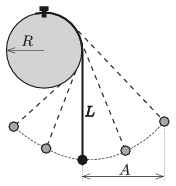(6 pont)

statistics## Problems with sign 'G'

Deadline expired on January 10, 2019.

G. 653. One morning two locomotives start from the engine shed and travel in the same direction. The first one is a diesel engine and has a speed of 90 km/h, whilst the other one is an electric engine, which started 1.5 minutes after the diesel engine, and has a speed of 20 m/s. 10 minutes after the diesel engine started it meets a fast train coming along the neighbouring track in the opposite direction. What is the speed of the fast train if it meets with the electric engine 1.5 minutes after it met with the diesel one?

(3 pont)

solution (in Hungarian), statistics

G. 654. The height of the thermal water of temperature $\displaystyle 30\;{}^\circ$C in a spa pool of base $\displaystyle 12~\mathrm{m}\times 20~\mathrm{m}$ is 75 cm. The pool was then filled with $\displaystyle 50\;{}^\circ$C water up to the height of 1 m. Due to heat loss the temperature of the mixture is $\displaystyle 2\;{}^\circ$C less than it would be without the loss. What amount of heat was lost during mixing the water?

(3 pont)

solution (in Hungarian), statistics

G. 655. A solid brick of mass 27 kg is placed onto a horizontal tabletop. If it is placed onto one of its face then the pressure on the tabletop is 4500 Pa. When another face is in contact with the table then the pressure is 7200 Pa, and facing down to its third side the pressure on the tabletop 2700 Pa. What is the density of the brick?

(4 pont)

solution (in Hungarian), statistics

G. 656. An old hair dryer has two switches. If the first switch is turned on then the hair dryer blows cold air. When the second switch is also turned on, then hot air is blown. When only the second switch is on, then neither the fan, nor the heating element are working. Draw a circuit diagram of the hair dryer.

(4 pont)

solution (in Hungarian), statistics## Problems with sign 'P'

Deadline expired on January 10, 2019.

P. 5078. In an ice-hockey match it is not rare that the speed of the hockey puck reaches the value of 160 km/h.

$\displaystyle a)$ How far could a puck having the above stated speed move on the ice if the coefficient of kinetic friction between the puck and the ice is 0.1?

$\displaystyle b)$ By what average force must the puck be shot in order to accelerate it to this speed? The stick and the puck are in contact for approximately 0.01 s. The weight of the puck is approximately 1.5 N.

One of the skaters of the local team – preparing for the penalty shot – starts to move with the puck. He decides that he will shoot the puck from a distance of 5 m from the goal line. He knows that the goal-tender of the opposing team has an excellent reaction time of 0.15 s.

$\displaystyle c)$ By what initial speed should he shoot the puck in order that it reaches the net before the goal-tender starts to move?

$\displaystyle d)$ By what force should he shoot the puck?

(4 pont)

solution (in Hungarian), statistics

P. 5079. Some plasticine balls, which have a hole through them and the same mass, can slide along a long straight rod. If the rod is in a slightly slant position the balls cannot start sliding, but if they are started they slide down with some acceleration. Gently starting the uppermost ball it reaches the ball below it. They stick and slide together. They hit the ball below them, stick to it and slide together, and so on. We can observe that each collision occurs at the same speed. What was the initial distance $\displaystyle L_n$ between the $\displaystyle n$-th and the $\displaystyle (n+1)$-st ball if the distance between the first two balls was $\displaystyle L_1$?

(5 pont)

solution (in Hungarian), statistics

P. 5080. By what percent does the average speed of the molecules of a sample of gas increase when the temperature of the gas is increased from $\displaystyle 27\;{}^\circ$C to $\displaystyle 159\;{}^\circ$C, if the gas is

$\displaystyle a)$ helium;

$\displaystyle b)$ hydrogen?

(3 pont)

solution (in Hungarian), statistics

P. 5081. What is the equivalent resistance across points $\displaystyle C$ and $\displaystyle D$ of the resistor system connected as shown in the figure, if each resistor has a resistance of $\displaystyle R$? By what percent does this equivalent resistance across $\displaystyle C$ and $\displaystyle D$ change if the resistor between points $\displaystyle A$ and $\displaystyle B$ is disconnected?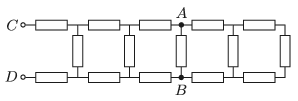(4 pont)

solution (in Hungarian), statistics

P. 5082. A small charged metal ball is suspended by a negligible-mass insulating thread of length $\displaystyle \ell=1$ m in uniform horizontal electric field. In the equilibrium position the angle between the thread and the vertical is $\displaystyle 30^\circ$. What is the period of the motion of the ball when it is displaced a bit from its equilibrium position?

(4 pont)

solution (in Hungarian), statistics

P. 5083. The angle of elevation of a slope is $\displaystyle \alpha$, the coefficient of friction on its surface is $\displaystyle \mu$. There is a small disc of mass $\displaystyle m$ and of charge $\displaystyle Q$ on the slope, and a uniform magnetic field of magnitude $\displaystyle B$ also exerts a force on the moving disc. The magnetic induction is perpendicular to the plane of the slope. The disc is released from rest. Determine the direction and the magnitude of the velocity of the disc, when the velocity becomes constant.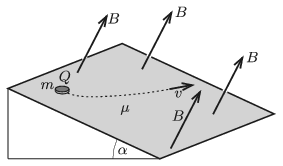(5 pont)

solution (in Hungarian), statistics

P. 5084. How does the wavelength of a light beam incident perpendicularly on a mirror change, if the mirror is moving at a speed of $\displaystyle v$ in the same direction as the direction of the propagation of the incident light beam?

$\displaystyle a)$ $\displaystyle v=150~\frac{\mathrm{m}}{\mathrm{s}}$;

$\displaystyle b)$ $\displaystyle v=150\,000~\frac{\mathrm{km}}{\mathrm{s}}$.

(5 pont)

solution (in Hungarian), statistics

P. 5085. The upper part of the figure (drawn to scale) shows how a light beam travels through a traditional converging lens. How would the light beam shown in the lower part of the figure travel after leaving the lens?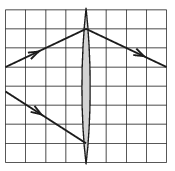(4 pont)

solution (in Hungarian), statistics

P. 5086. How much energy is needed to split the nucleus of an oxygen into four alike parts? What is the minimum energy of a neutron, which is able to split the – initially stationary – nucleus of the oxygen?

(4 pont)

solution (in Hungarian), statistics

P. 5087. Is it theoretically possible to spot by the naked eye a crater of diameter of 80 km on the surface of the Moon if the diameter of our pupil is 5 mm?(4 pont)

solution (in Hungarian), statistics

P. 5088. The bob of a simple pendulum of length $\displaystyle \ell$ is displaced horizontally and then released. When the thread reaches the vertical position, it hits a nail and from that moment onward only the lower part of the thread of length $\displaystyle r$ is moving.

What is the ratio of $\displaystyle r/\ell$, if the bob leaves the circular orbit somewhere in its upward motion, and then moving freely it exactly bumps into the nail?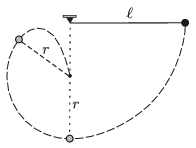(6 pont)

solution (in Hungarian), statistics